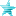Started by FoQuS, October 30, 2007, 08:45:01 PM

#### Cyanide#21  November 04, 2007, 01:40:42 AM
••• Legendary XIII
• I am the eye of the storm to come!
You're changing explod as you travel up the power settings. You need to remove the old one when you hit a new setting.

Creation triggers are power > 1000, power = [1000,1999] and power = [2000,3000]

Give each explod an unique ID and remove it when power > 1000/2000

All same state.In M.U.G.E.N there is no magic button

They say a little knowledge is a dangerous thing, but it's not one half so bad as a lot of ignorance.

#### FoQuS#22  November 13, 2007, 11:05:37 PM
••[Statedef 1000]
Type =S
physics = S
anim = 1000
ctrl = 0
velset = 0,0

trigger1 = ((GameTime%3) = 0) && (command = "hold_x") && (command = "hold_a")
value = 25

[State 1000, Explod]
type = Explod
;trigger1 = animelem = 4
trigger1 = power > 2000
;trigger1 = (command = "hold_x") && (command = "hold_a")
;trigger1 = command = "hold"
trigger1 = time = 0
anim = 1100
ID = 1100
pos = 0,0
postype = p1  ;p2,front,back,left,right
facing = 1
vfacing = 1
bindtime = 1
vel = 0,0
accel = 0,0
random = 0,0
removetime = -1
;supermove
;ausemove
scale = 1,1
sprpriority = 3
ontop = 1
ownpal = 1
removeongethit = 0

[State 1001, RemoveExplod]
type = RemoveExplod
trigger1 = (command != "hold_x") || (command != "hold_a")
id =  1100

[State 1000, Explod]
type = Explod
trigger1 = animelem = 4
trigger1 = power <= 2000
anim = 6030;
ID = 6030;
pos = 0,0;-110,-190
postype = p1  ;p2,front,back,left,right
facing = 1
vfacing = 1
bindtime = 1
vel = 0,0
accel = 0,0
random = 0,0
removetime = -1
supermove
pausemove
scale = 1,1
sprpriority = 2
ontop = 0
ownpal = 0
removeongethit = 0
;ignorehitpause =
;persistent =

[State 1000, RemoveExplod]
type = RemoveExplod
trigger1 = time = 0
trigger1 = power > 2000
id = 6030;Var(9)
;ignorehitpause =
;persistent =

[State 1000, Explod2]
type = Explod
trigger1 = AnimElem = 4
trigger1 = power >= 4000
anim = 6192
ID = 6192
pos = -60,60
postype = p1  ;p2,front,back,left,right
facing = 1
vfacing = 1
bindtime = 1
vel = 0,0
accel = 0,0
random = 0,0
removetime = -1
;supermove
;ausemove
scale = 1,1
sprpriority = 2
ontop = 0
ownpal = 1
removeongethit = 0

[State 1000, Explod]
type = Explod
trigger1 = animelem = 4
anim = 6031
ID = 6031
pos = 0,3
postype = p1  ;p2,front,back,left,right
facing = 1
vfacing = 1
bindtime = 1
vel = 0,0
accel = 0,0
random = 0,0
removetime = -1
scale = 1,1
sprpriority = 0
ontop = 0
ownpal = 0
removeongethit = 0
;ignorehitpause =
;persistent =

[State 1000, EnvShake]
type = EnvShake
trigger1 = time > 44
trigger1 = TimeMod = 4,0
time = 4
freq = 60
ampl = -4
;phase = 90
;ignorehitpause =
persistent = 1

[State 1000, PlaySnd]
type = PlaySnd
trigger1 = Time = 0
channel = 1
value = 1100, 0

[State 1000, PlaySnd]
type = PlaySnd
trigger1 = Time = 0
channel = 1
value = 1100, 2
loop = 1

[State 1000, PlaySnd]
type = PlaySnd
trigger1 = time = 44
channel = 2
value = 1100, 1
loop = 80

[State 1000, End]
type = ChangeState
trigger1 = (command != "hold_x") || (command != "hold_a")
trigger2 = power = 2000
trigger3 = power = 4000
value = 1001

[State 1000, End]
type = null;ChangeState
trigger1 = (command != "hold_x") || (command != "hold_a")
trigger2 = power = 4000
value = 1001

#### Cyanide#23  November 13, 2007, 11:37:34 PM
••• Legendary XIII
• I am the eye of the storm to come!
You have 2 removeexplods. 1 will only trigger if you release X or Y, the other one only triggers when you enter the state.

You need 1 removeexplod, per explod, with the conditions it should be removed under.

For example the level 1 version should always be removed when power > 1000 OR when you release the keys.In M.U.G.E.N there is no magic button

They say a little knowledge is a dangerous thing, but it's not one half so bad as a lot of ignorance.